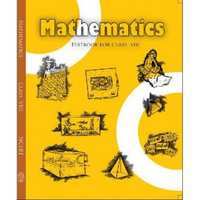# NCERT solutions for Class 8 Maths chapter 4 - Practical Geometry [Latest edition]

#### Chapters## Chapter 4: Practical Geometry

Exercise 4.1Exercise 4.2Exercise 4.3Exercise 4.4Exercise 4.5
Exercise 4.1 [Page 60]

### NCERT solutions for Class 8 Maths Chapter 4 Practical GeometryExercise 4.1 [Page 60]

Exercise 4.1 | Q 1.1 | Page 60

AB = 4.5 cm

BC = 5.5 cm

CD = 4 cm

AC = 7 cm

Exercise 4.1 | Q 1.2 | Page 60

JU = 3.5 cm

UM = 4 cm

MP = 5 cm

PJ = 4.5 cm

PU = 6.5 cm

Exercise 4.1 | Q 1.3 | Page 60

Parallelogram MORE

OR = 6 cm

RE = 4.5 cm

EO = 7.5 cm

Exercise 4.1 | Q 1.4 | Page 60

Rhombus BEST

BE = 4.5 cm

ET = 6 cm

Exercise 4.2 [Page 62]

### NCERT solutions for Class 8 Maths Chapter 4 Practical GeometryExercise 4.2 [Page 62]

Exercise 4.2 | Q 1.1 | Page 62

LI = 4 cm

IF = 3 cm

TL = 2.5 cm

LF = 4.5 cm

IT = 4 cm

Exercise 4.2 | Q 1.2 | Page 62

OL = 7.5 cm

GL = 6 cm

GD = 6 cm

LD = 5 cm

OD = 10 cm

Exercise 4.2 | Q 1.3 | Page 62

Rhombus BEND

BN = 5.6 cm

DE = 6.5 cm

Exercise 4.3 [Page 64]

### NCERT solutions for Class 8 Maths Chapter 4 Practical GeometryExercise 4.3 [Page 64]

Exercise 4.3 | Q 1.1 | Page 64

MO = 6 cm

OR = 4.5 cm

∠M = 60°

∠O = 105°

∠R = 105°

Exercise 4.3 | Q 1.2 | Page 64

PL = 4 cm

LA = 6.5 cm

∠P = 90°

∠A = 110°

∠N = 85°

Exercise 4.3 | Q 1.3 | Page 64

Parallelogram HEAR

HE = 5 cm

EA = 6 cm

∠R = 85°

Exercise 4.3 | Q 1.4 | Page 64

Rectangle OKAY

OK = 7 cm

KA = 5 cm

Exercise 4.4 [Page 67]

### NCERT solutions for Class 8 Maths Chapter 4 Practical GeometryExercise 4.4 [Page 67]

Exercise 4.4 | Q 1.1 | Page 67

DE = 4 cm

EA = 5 cm

AR = 4.5 cm

∠E = 60°

∠A = 90°

Exercise 4.4 | Q 1.2 | Page 67

TR = 3.5 cm

RU = 3 cm

UE = 4 cm

∠R = 75°

∠U = 120°

Exercise 4.5 [Page 68]

### NCERT solutions for Class 8 Maths Chapter 4 Practical GeometryExercise 4.5 [Page 68]

Exercise 4.5 | Q 1 | Page 68

Draw the following:

The square READ with RE = 5.1 cm

Exercise 4.5 | Q 2 | Page 68

Draw the following:

A rhombus whose diagonals are 5.2 cm and 6.4 cm long.

Exercise 4.5 | Q 3 | Page 68

Draw the following:

A rectangle with adjacent sides of length 5 cm and 4 cm.

Exercise 4.5 | Q 4 | Page 68

Draw the following:

A parallelogram OKAY where OK = 5.5 cm and KA = 4.2 cm.

## Chapter 4: Practical Geometry

Exercise 4.1Exercise 4.2Exercise 4.3Exercise 4.4Exercise 4.5## NCERT solutions for Class 8 Maths chapter 4 - Practical Geometry

NCERT solutions for Class 8 Maths chapter 4 (Practical Geometry) include all questions with solution and detail explanation. This will clear students doubts about any question and improve application skills while preparing for board exams. The detailed, step-by-step solutions will help you understand the concepts better and clear your confusions, if any. Shaalaa.com has the CBSE Class 8 Maths solutions in a manner that help students grasp basic concepts better and faster.

Further, we at Shaalaa.com provide such solutions so that students can prepare for written exams. NCERT textbook solutions can be a core help for self-study and acts as a perfect self-help guidance for students.

Concepts covered in Class 8 Maths chapter 4 Practical Geometry are Constructing a Quadrilateral When the Lengths of Four Sides and a Diagonal Are Given, Constructing a Quadrilateral When Two Diagonals and Three Sides Are Given, Constructing a Quadrilateral When Two Adjacent Sides and Three Angles Are Known, Constructing a Quadrilateral When Three Sides and Two Included Angles Are Given, Some Special Cases, Introduction to Practical Geometry.

Using NCERT Class 8 solutions Practical Geometry exercise by students are an easy way to prepare for the exams, as they involve solutions arranged chapter-wise also page wise. The questions involved in NCERT Solutions are important questions that can be asked in the final exam. Maximum students of CBSE Class 8 prefer NCERT Textbook Solutions to score more in exam.

Get the free view of chapter 4 Practical Geometry Class 8 extra questions for Class 8 Maths and can use Shaalaa.com to keep it handy for your exam preparation